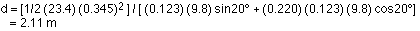## Applying energy concepts

10-15-99

Section 6.9

With energy, we've got another tool in our physics toolbox to use to attack problems. Let's try some more examples to see how these energy concepts are applied.

### Air resistance

Air resistance can be a tricky thing to account for. Generally, the force applied by air to a moving object is proportional to the square of the speed of the object. We know how to handle constant forces, but a force that depends on speed is a different story. If we apply energy to the problem, however, we can make some headway.

Take, for instance, a simple coffee filter. It's very light, and has a relatively large surface area. If you let a coffee filter fall, it falls at roughly constant speed; this is because the force of gravity is balanced by the force of air resistance. Note that the constant speed is known as the terminal velocity - the same kind of thing applies to skydivers. Let's say we drop a coffee filter (from rest) from a height of 1.5 m. If there was no air resistance, the filter would take about 0.55 seconds to hit the ground, and be traveling at a final speed of 5.4 m/s.

The actual time for a coffee filter to fall 1.5 m is more like 1.5 seconds (try timing it yourself). Doing some estimating, making the assumption that the speed is constant over this time interval gives a speed of 1.0 m/s. How much work is done by air resistance? Approximately what is the force of air resistance on the filter?

Applying conservation of energy makes it easy to determine the work done. This is as long as the mass is known - weighing a stack of filters on a balance, I found that one filter weighs approximately 1 gram. This gives the coffee filter an initial potential energy, relative to the floor, of 0.001 x 1.5 x 9.8 which is approximately 0.015 J. When it hits the ground the kinetic energy is 0.0005 J. Clearly, energy has been lost - in fact, almost all the energy has been lost! The work done by friction equals the change in energy, so it's almost -0.015 J. It's negative because it opposes the motion: the displacement is down and the force is up.

The work is the force times the displacement. The average force in this case is therefore about 0.01 N. This is the same answer you get if you set the gravitational force equal to the force of air resistance, so that's a good sign that we're doing things correctly.

### An example, using the PE of a spring

A 0.123 kg block sits on a plane inclined at 20°. The block is pushed back against a spring (k = 23.4 N / m), compressing the spring by 0.345 m. When the block is let go, it is accelerated up the incline by the spring. The coefficient of kinetic friction between the block and the incline is 0.220.

How far up the incline does the block go?

Attack this problem using work and energy. The initial energy (stored in the spring) is equal to the final energy (gravitational PE) plus whatever gets lost to friction. Writing this as an equation gives:

Energy before = energy after + energy lost to friction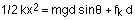A free-body diagram tells us that: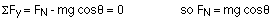Substituting this in to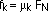transforms the energy equation to: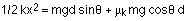Solving this for d, the distance the block travels up the slope, gives: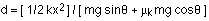Plugging in all the numbers gives: